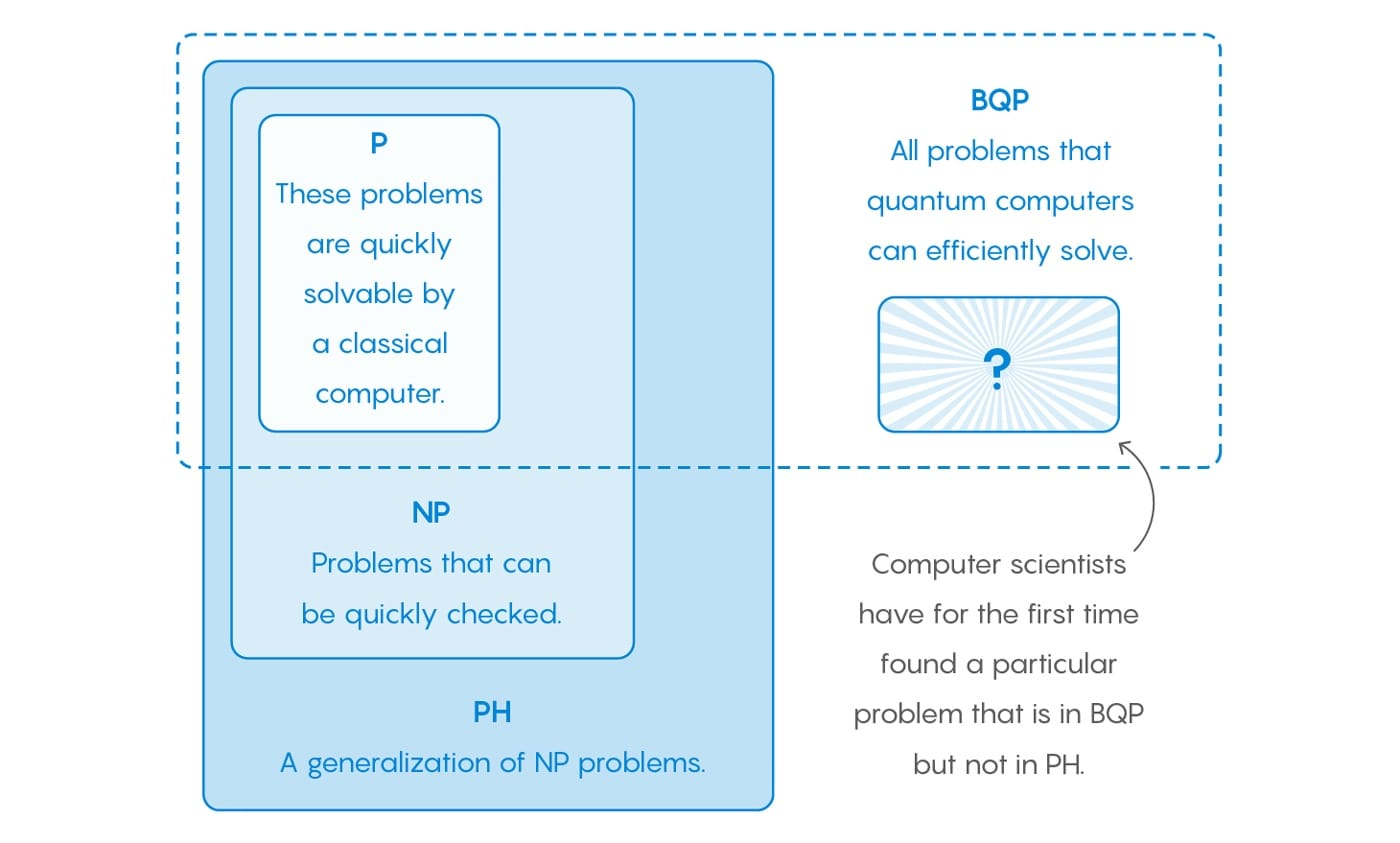Over the past couple of years, I’ve been investigating Quantum Computing. I have previously written two articles, covering an overview or the technology, hardware requirements, value proposition and the commercial model.

Similar to Blockchain, Quantum Computing is a complex technology that is largely misunderstood. As a result, I am always looking for new resources that tangibly explain its potential.

Earlier this week, Seeker published a new eight-minute video, which does a great job of explaining the technology and highlights the drug discovery opportunity.

One common misconception is that Quantum Computing will outperform Classical Computing at every task. In reality, Quantum Computing targets a specific set of tasks, which are categorized on the diagram below:As you can see, scientists have identified a class of problem known as Bounded-error Quantum Polynomial (BQP), which can only be solved by Quantum Computing.

To better understand BQP, it is important to recognise the different complexity classes. Quantum Magazine recently posted a good article, which provides a high-level overview of the complexity classes. I have attempted to summarise the key points below.

### Complexity Classes

A basic task of theoretical computer science is to sort problems into complexity classes. A complexity class contains all problems that can be solved within a given resource budget, where the resource is something like time or memory.

#### P = Polynomial

• Short Description: All problems that are easy for a Classical Computer to solve.

• Example: Typical problems include, identifying the shortest path between two points.

#### NP = Nondeterministic Polynomial

• Short Description: All problems that can be quickly verified by a Classical Computer once a solution is given.

• Example: The “Traveling Salesman Problem”. Given a list of cities and the distances between each pair of cities, what is the shortest possible route that visits each city and returns to the origin city?

#### PH = Polynomial Hierarchy

• Short Description: A generalisation of NP. It contains all the problems you get if you start with a problem in NP and add additional layers of complexity.

• Example: Determine if there exists a clique of size 50 but no clique of size 51.

#### PSPACE = Polynomial Space

• Short Description: Does not place a focus on time, instead it contains all the problems that can be solved with a reasonable amount of memory.

• Example: Every problem in P, NP, PH, and PS.

#### BQP = Bounded-error Quantum Polynomial Time

• Short Description: All problems that are easy for a Quantum Computer to solve.

• Example: Identify the prime factors of an integer.

#### EXP = Exponential

• Short Description: All the problems that can be solved in an exponential amount of time by a Classical Computer.

• Example: Contains all the previous classes (e.g. P, NP, PH, PSPACE, and BQP), however, problems categorised as EXP are not in P.

#### BPP = Bounded-error Probabilistic Polynomial Time

• Short Description: Problems that can be quickly solved by algorithms that include an element of randomness.

• Example: Two different formulas that each produce P, that have many variables. Do the formulas compute the exact same P? This is called the “Polynomial Identity Testing” problem.

### Conclusion

Quantum Computing remains a fascinating area, which continues to challenge human understanding and therefore is in a constant state of evolution.

For example, BQP was first defined in 1993, encompassing all problems that can be solved by Quantum Computing.

Since then, computer scientists have hoped to contrast BQP with a class of problems known as PH, which encompasses all problems that can be solved by a Classical Computer. This required finding a problem that could be proven to be in BQP, but not in PH.

This breakthrough was achieved in May 2018, by computer scientists Ran Raz and Avishay Tal. Their findings were documented in the paper “Oracle Separation of BQP and PH”.

In my opinion, further breakthroughs in Quantum Mechanics are limited by the current level of software abstraction, which itself is limited by the hardware.

For example, future Quantum Computing hardware breakthroughs, such as Fault Tolerance and Coherence, will be followed quickly by greater software abstraction. This will result in scientists being able to identify and address increasingly complex problems.

This can be compared with the field of astronomy, which did not see major breakthroughs until after the creation of the telescope.